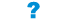# Extension:WikiQuizEXTENSIONS

WikiQuiz is an extension that allows interactive quizzes to be created. The quiz questions are multiple choice (five options) with conditional feedback given.

To write a quiz without using any code, use the extension QuizWriter found here.

## Display

The question number is located to the left of the question.

The question is in bold with the five choices displayed below it.

Each choice can be selected by "checking" a radio button to the left of the choice.

The user checks the choice by pressing the "Reveal Feedback" button.

Depending on the user's choice, different feedback will be given. Different feedback can be written for each choice.

• If no choice is selected the user will be prompted to select a choice with the text with a blue background.
• If the user selects the correct choice, feedback is displayed on a pale green back ground.
• If the wrong choice is selected the feedback is displayed on a pale red background.

After clicking "Reveal Feedback" the user is able to re-click to try a different choice.

To see how this extension function experiment with the example below.

## Syntax

 ``` ```

### Syntax Explanation

Parameter Input Output
questionnumber Numeric Determines question number. Question number must be set if there is more than one question on a page otherwise feedback will not be given.
question Alphanumeric The question that is being asked.
choice1-5 Alphanumeric Potential choices for the question that appears alongside the radio buttons.
correctchoice Numeric value (1-5)
Determines which choice is correct
'1' corresponding to 'choice1' etc.
Determines which choice to the question is correct.
feedback1-5 Alphanumeric input (with wikimarkup)
'feedback1' corresponds with 'choices1' etc.
Gives feedback for the choices.
image Page name of image required Gives an image to the right of the questions choices.
Image size is fixed.
If the image parameter is not defined the default image Image:WikiQuiz_NoImage.png is loaded.

## Example

 ``` ```

### Gives

1

A 36kg dog is to undergo a bitch spey and she is to receive fluid therapy at the surgical rate. Calculate how many drops per second she should receive given that the giving set administers 20 drops per ml.

 2 drops per second1 drop per second 0.1 drops per second 0.4 drops per second 6 drops per second Please select an option Correct!The surgical fluid rate is five times maintenance i.e. 10mls/kg/hr. Since the dog is 36kg, it will require 360 mls/hr, which is 6 mls/min (divide 360 by 60) and 0.1 mls/sec (divide 6 by 60). Then multiply 0.1 by the giving set factor which is 20 drops per ml to get 2 drops/sec. WikiVet Article:Fluid Rate Calculations Incorrect! Have you used a fluid rate of 5mls/kg/hr? Remember, surgical fluid rate is five times maintenance i.e. 10mls/kg/hr. Since the dog is 36kg, it will require 360 mls/hr, which is 6 mls/min (divide 360 by 60) and 0.1 mls/sec (divide 6 by 60). Then multiply 0.1 by the giving set factor which is 20 drops per ml to get 2 drops/sec. WikiVet Article:Fluid Rate Calculations Incorrect! Have you forgotten to multiply by the giving set factor? The surgical fluid rate is five times maintenance i.e. 10mls/kg/hr. Since the dog is 36kg, it will require 360 mls/hr, which is 6 mls/min (divide 360 by 60) and 0.1 mls/sec (divide 6 by 60). Then multiply 0.1 by the giving set factor which is 20 drops per ml to get 2 drops/sec. WikiVet Article:Fluid Rate Calculations Incorrect! Have you used maintenance fluid rate i.e. 2mls/kg/hr? The surgical fluid rate is five times maintenance i.e. 10mls/kg/hr. Since the dog is 36kg, it will require 360 mls/hr, which is 6 mls/min (divide 360 by 60) and 0.1 mls/sec (divide 6 by 60). Then multiply 0.1 by the giving set factor which is 20 drops per ml to get 2 drops/sec. WikiVet Article:Fluid Rate Calculations Incorrect! Have you forgotten to divide by 60 twice and multiply by the giving set factor? The surgical fluid rate is five times maintenance i.e. 10mls/kg/hr. Since the dog is 36kg, it will require 360 mls/hr, which is 6 mls/min (divide 360 by 60) and 0.1 mls/sec (divide 6 by 60). Then multiply 0.1 by the giving set factor which is 20 drops per ml to get 2 drops/sec. WikiVet Article:Fluid Rate Calculations